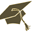Thesis (Selection of subject)(version: 285)
Assignment details
Login via CASAritmetika číselných těles a zobecněné řetězové zlomky
Thesis title in Czech: Aritmetika číselných těles a zobecněné řetězové zlomky Arithmetics of number fields and generalized continued fractions 2016/2017 dissertation Department of Algebra (32-KA) Mgr. Vítězslav Kala, Ph.D. hidden- assigned and confirmed by the Study Dept. 20.09.2017 20.09.2017 03.10.2017
 Guidelines The aim of the thesis is to study the arithmetics of number fields, in particular the structure of totally positive additively indecomposable integers. In the case of real quadratic fields, these can be nicely characterized in terms of periodic continued fractions, which then has applications to the study of universal quadratic forms. However, the classical theory no longer works for higher degree number fields, so the student will investigate and develop other methods based on generalized continued fractions or numeration systems, e.g., the Jacobi-Perron algorithm or continued fractions with algebraic coefficients.
 References Valentin Blomer and Vitezslav Kala, Number fields without n-ary universal quadratic forms, Math. Proc. Cambridge Philos. Soc. 159 (2015), no. 2, 239–252. Vítezslav Kala, Norms of indecomposable integers in real quadratic fields, J. Number Theory 166 (2016), 193-207. Vítezslav Kala, Universal quadratic forms and elements of small norm in real quadratic fields, Bull. Aust. Math. Soc. 94 (2016), 7-14. Fritz Schweiger, Multidimensional Continued Fractions, Oxford University Press, 2000. Julien Bernat, Continued fractions and numeration in the Fibonacci base, Discrete Math. 306 (2006), no. 22, 2828–2850. Leon Bernstein, The Jacobi-Perron Algorithm Its Theory and Application, Lecture Notes in Mathematics, Volume 207 (1971).

Charles University | Information system of Charles University | http://www.cuni.cz/UKEN-329.html